# Forces air resistance force contact force noncontact force

• Slides: 17Forces • air resistance • force • contact force • noncontact force • gravity • friction • Newton’s first law of motion • Newton’s second law of motion • Newton’s third law of motionWhat is force? • A force is a push or a pull on an object. • Force has both size and direction. • You can use arrows to show the size and direction of a force. • The unit force is the newton (N).What is force? (cont. ) • A contact force is a push or a pull on one object by another object that is touching it. • A force that one object can apply to another object without touching it is a noncontact force.Gravity—A Noncontact Force • Gravity is an attractive force that exists between all objects that have mass. • The size of a gravitational force depends on the masses of the objects and the distance between them.Gravity—A Noncontact Force (cont. ) If the mass of an object increases, the gravitational force increases between it and another object.Gravity—A Noncontact Force (cont. ) As two objects move apart, the gravitational force between them decreases.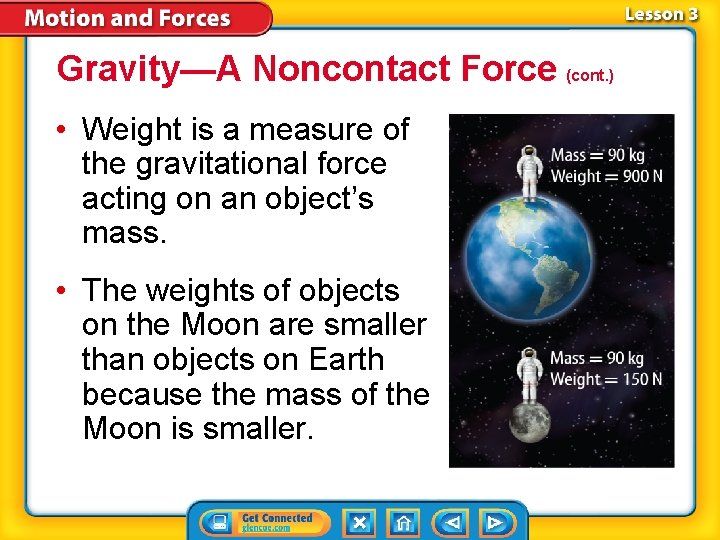Gravity—A Noncontact Force (cont. ) • Weight is a measure of the gravitational force acting on an object’s mass. • The weights of objects on the Moon are smaller than objects on Earth because the mass of the Moon is smaller.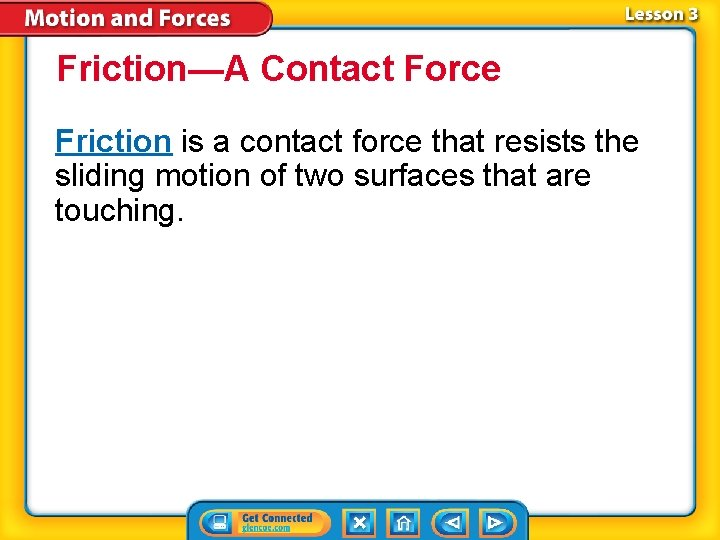Friction—A Contact Force Friction is a contact force that resists the sliding motion of two surfaces that are touching.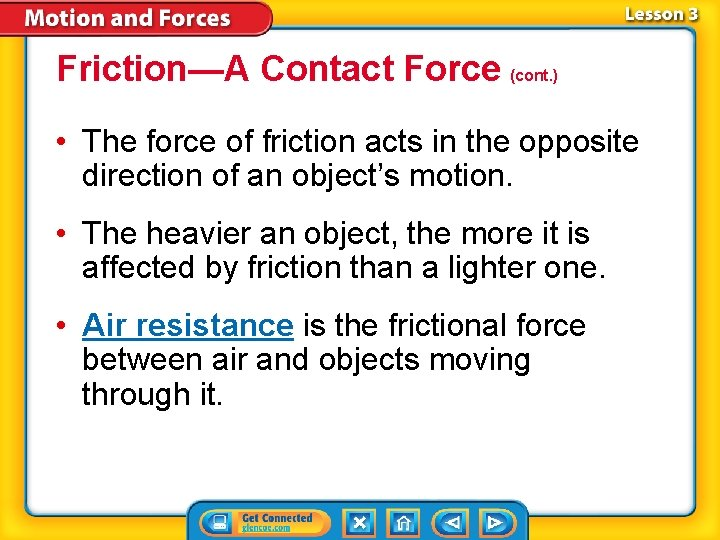Friction—A Contact Force (cont. ) • The force of friction acts in the opposite direction of an object’s motion. • The heavier an object, the more it is affected by friction than a lighter one. • Air resistance is the frictional force between air and objects moving through it.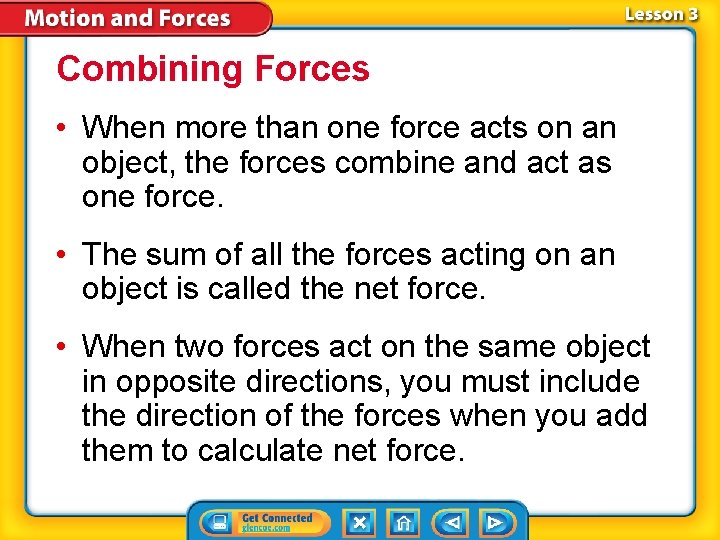Combining Forces • When more than one force acts on an object, the forces combine and act as one force. • The sum of all the forces acting on an object is called the net force. • When two forces act on the same object in opposite directions, you must include the direction of the forces when you add them to calculate net force.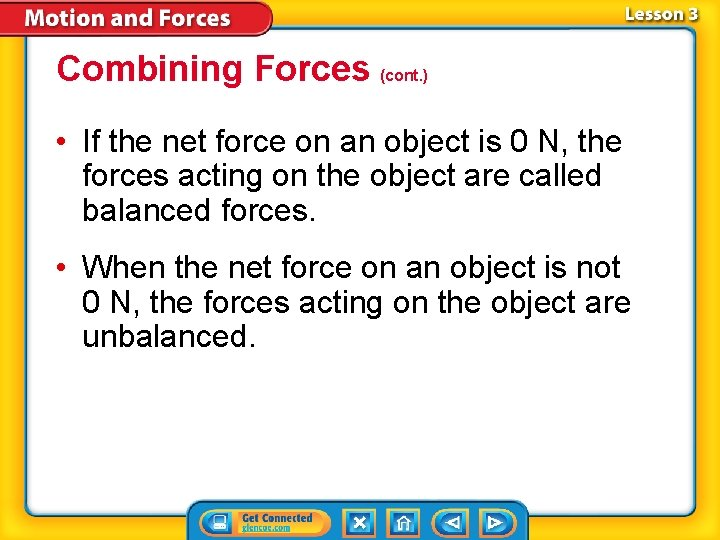Combining Forces (cont. ) • If the net force on an object is 0 N, the forces acting on the object are called balanced forces. • When the net force on an object is not 0 N, the forces acting on the object are unbalanced.Unbalanced Forces and Acceleration • When unbalanced forces act on an object, the object’s velocity changes. • Unbalanced forces can change either the speed or the direction of motion.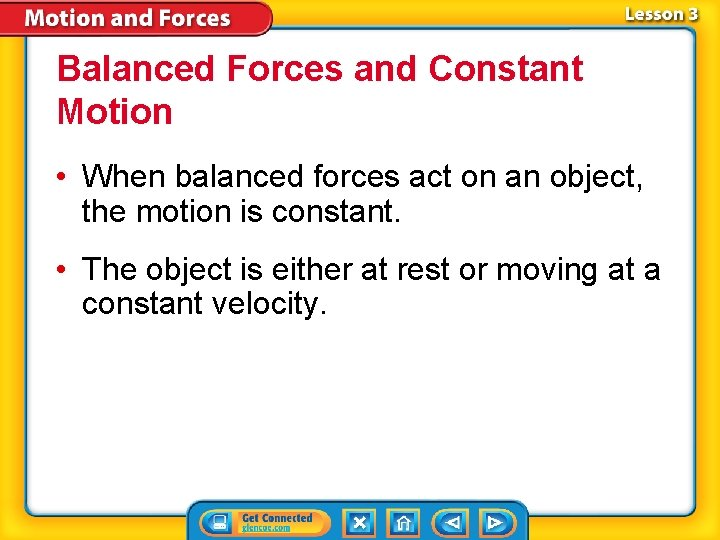Balanced Forces and Constant Motion • When balanced forces act on an object, the motion is constant. • The object is either at rest or moving at a constant velocity.Forces and Newton’s Laws of Motion • According to Newton’s first law of motion, if the net force acting on an object is zero, the motion of the object does not change. • Inertia is the tendency of an object to resist a change in its motion.Forces and Newton’s Laws of Motion (cont. ) According to Newton’s second law of motion, the acceleration of an object is equal to the net force exerted on the object divided by the object’s mass.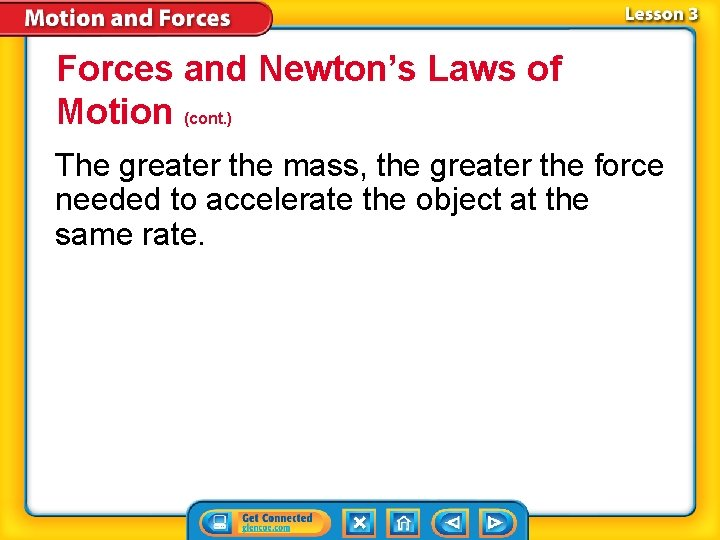Forces and Newton’s Laws of Motion (cont. ) The greater the mass, the greater the force needed to accelerate the object at the same rate.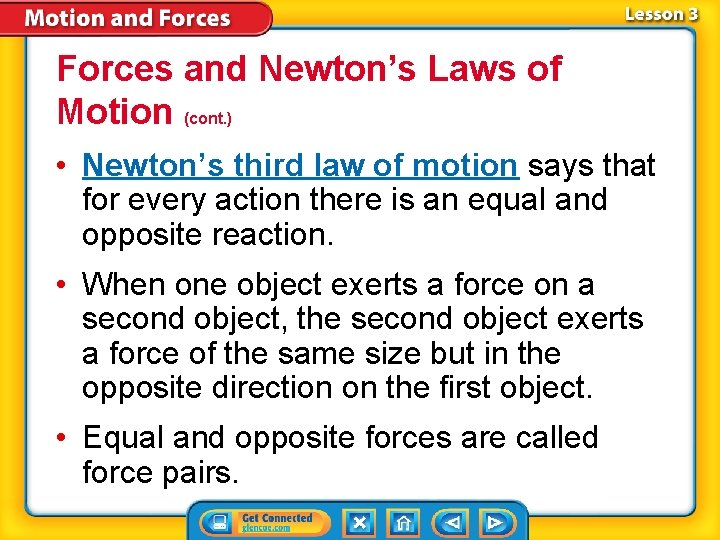Forces and Newton’s Laws of Motion (cont. ) • Newton’s third law of motion says that for every action there is an equal and opposite reaction. • When one object exerts a force on a second object, the second object exerts a force of the same size but in the opposite direction on the first object. • Equal and opposite forces are called force pairs.